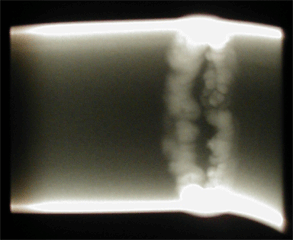105 Questions | Attempts: 3716SettingsIndustrial Radiography quiz for people studying for RT 2 CGSB exam.

• 1.
Who is given credit for the discovery of X-ray?
• A.

Henri Becquerel

• B.

Wilhelm Roentgen

• C.

Marie Curie

• D.

Pierre Curie

• 2.
There are four types of radiation-matter interactions that can contribute to the total attenuation. These are:
• A.

Compton scattering, pair production, photoelectric absorption, rayleigh scattering

• B.

Compton scattering, electron exchange, photoelectric absorption, rayleigh scattering

• C.

Electron exchange, pair production, photoelectric absorption, rayleigh scattering

• D.

None of the above

• 3.
X-rays and Gamma rays are a form of:
• A.

Light

• B.

• C.

• D.

Both B and C

• 4.
Who is given credit for the discovery of radioactive materials?
• A.

Henri Becquerel

• B.

Wilhelm Roentgen

• C.

Marie Curie

• D.

Pierre Curie

• 5.
A specific radioactive source will always produce gamma rays at the same:
• A.

Intensity

• B.

Activity

• C.

Energy Levels

• D.

None of the above

• 6.
Higher energy radiation will have more:
• A.

Speed

• B.

Incident Intensity

• C.

Penetrating Power

• D.

Both B and C

• 7.
X-rays and Gamma rays present a health risk because they are a form of ionizing radiation, which means that the radiation has enough energy to:
• A.

Vibrate water molecules and generate heat

• B.

Break chemical bonds

• C.

Break physical bonds

• D.

None of the above

• 8.
X-rays and Gamma rays have significant penetrating power due to their:
• A.

Short Wavelength

• B.

Medium Wavelength

• C.

Long Wavelength

• D.

Wide range of wavelengths

• 9.
Ionizing radiation can be used in industrial radiography because the health hazards:
• A.

Have been eliminated with controls and procedures

• B.

Are minimized through controls and procedures

• C.

Are worth the risk

• D.

Are being ignored

• 10.
X-rays and Gamma rays:
• A.

Always travel in a straight line

• B.

Can be influenced by an electrical field

• C.

Can be influenced by a magnetic field

• D.

None of the above

• 11.
X-rays and Gamma rays are often referred to as photons because:
• A.

They possess a charge

• B.

They have mass

• C.

They occur as small packets of energy

• D.

None of the above

• 12.
X-rays and Gamma rays:
• A.

Are both affected by radioactive decay

• B.

Are both produced by a radioactive atom

• C.

Have completely different properties

• D.

Differ only in their source

• 13.
Newtons Inverse Square Law is useful in radiography because it indicates how the radiation intensity is affected by:
• A.

• B.

Distance from the source

• C.

The size of the source

• D.

None of the above

• 14.
The rate at which a radioactive isotope changes to a more stable atom is know as the:
• A.

Isotope decay rate

• B.

Half-life

• C.

Activity

• D.

Attenuation

• 15.
Attenuation of radiation is due to:
• A.

Absorption

• B.

Scattering

• C.

• D.

Both A and B

• 16.
The number of X-ray or Gamma photons that are transmitted through a material depends on the:
• A.

Energy of the photons

• B.

Thickness of the material

• C.

Atomic number of the material

• D.

All of the above

• 17.
After traveling through two half-value layers, the incident radiation has been reduced to:
• A.

50%

• B.

35%

• C.

20%

• D.

None of the above

• 18.
The thickness of any given material where 50% of the incident energy has been attenuated is known as the:
• A.

Half-value layer

• B.

Linear attenuation coefficient

• C.

Decay rate

• D.

Mass attenuation coefficient

• 19.
Undercut is the loss of resolution at a sharp, thickness transition area due to:
• A.

Scattering within the part

• B.

Backscatter

• C.

Sidescatter

• D.

Scattering within the film

• 20.
Unexposed X-ray film is comprised of a plastic, transparent base coated with an emulsion containing radiation-sensitive particle known as:
• A.

Metalic silver crystals

• B.

Silver halide grains

• C.

Both A and B

• D.

Neither A and B

• 21.
Collimators are used to:
• A.

• B.

• C.

Increase film latitude

• D.

Decrease film latitude

• 22.
Bremsstrahlung production of X-rays produces radiation that is composed of:
• A.

A small number of very defined energies

• B.

A continuous spectrum of energies over some range

• C.

• D.

None of the above

• 23.
Exposure to ionizing radiation can be limited:
• A.

With the use of shielding

• B.

By increasing distance form the source

• C.

By limiting the time exposed to the radiation

• D.

All of the above

• 24.
• A.

Bremsstrahlung processes

• B.

K-shell emmission processes

• C.

• D.

Both A and B

• 25.
• A.

By splitting the nucleus of atoms in the source material

• B.

Adding electrons to the source material

• C.

Introducing an extra neutron to the atoms of the source material

• D.

None of the above

## Related TopicsBack to top
×

Wait!
Here's an interesting quiz for you.